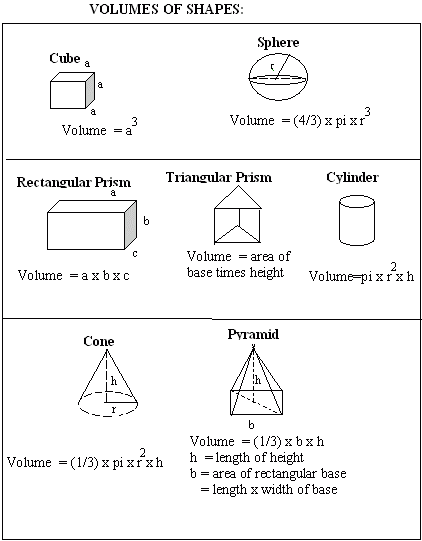Name: ___________________Date:___________________

 Volume is a measure of how much space a space figure takes up. Volume is used to measure a space figure just as area is used to measure a plane figure. Directions: Review the following table and answer the questions.Examples: The volume of a cube with side length 4 is 4 x 4 x 4 = 64. The volume of a rectangular prism with length 5, width 4 and height 2 is 5 x 4 x 2 = 40. The volume of a cylinder with radius 2 and height 4 is pi x 2 x 2 x 4 = 16 pi. The volume of a triangular prism with area of triangular base 8 and height 5 is 8 x 5 = 40. The volume of a cone with radius 2 and height 9 is pi x 2 x 2 x 9 x 1/3 = 12 pi. The volume of a sphere of radius 6 is pi x 6 x 6 x 6 x 4/3 = 288 pi. Directions: Answer the following questions. Also write at least 2 examples to find the volume of each kind of figure.